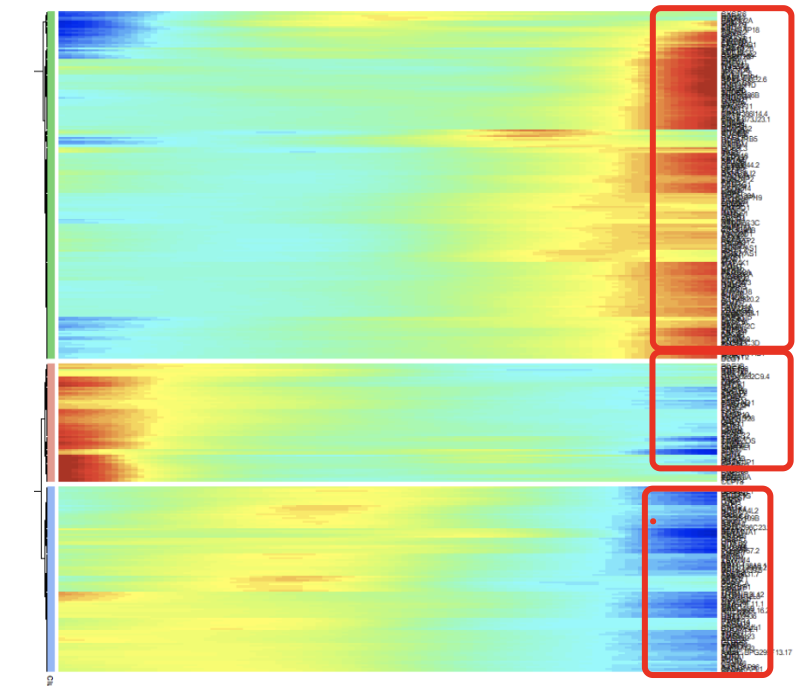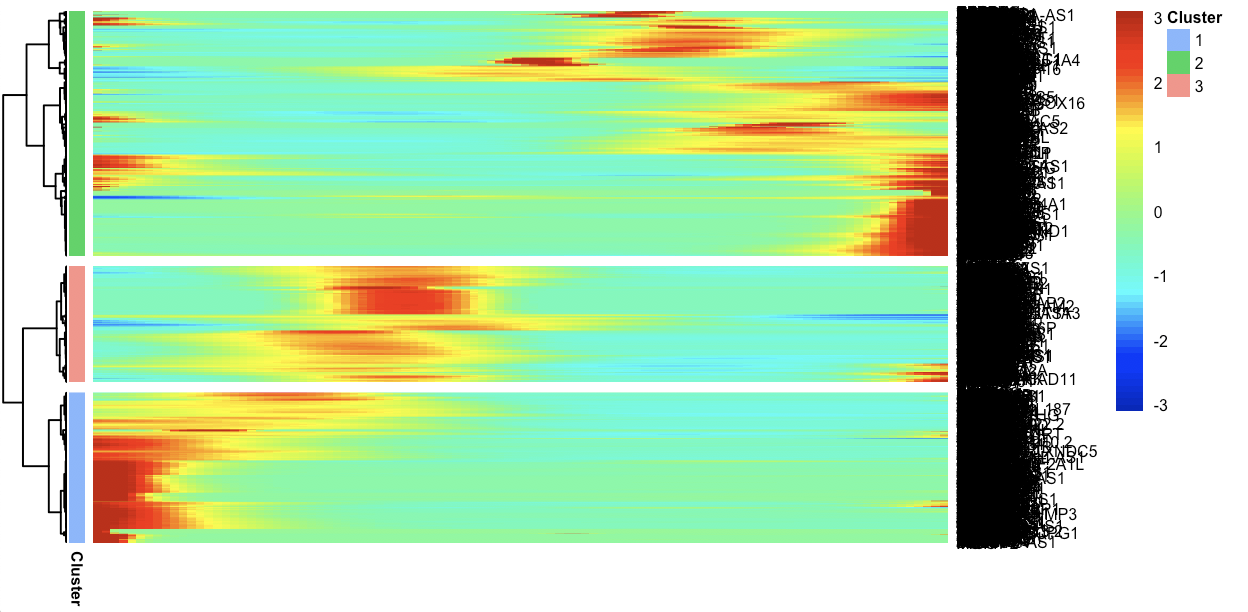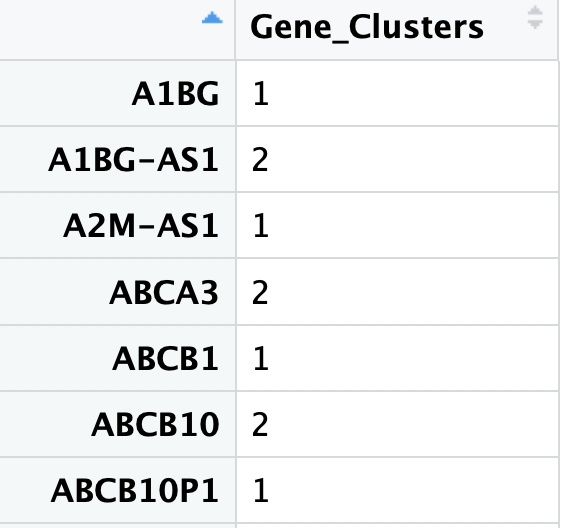# 拟时序分析的热图提取基因问题### 首先根据细胞发育谱系来绘制热图

``````plot_cell_trajectory(cds, color_by = "Biological_Condition")
# 可以很明显看到细胞的发育轨迹
plot_cell_trajectory(cds, color_by = "State")
plot_cell_trajectory(cds, color_by = "Pseudotime")
plot_cell_trajectory(cds, color_by = "State") +
facet_wrap(~State, nrow = 1)
``````

``````plot_pseudotime_heatmap(cds[ordering_genes,],
num_clusters = 3,
cores = 1,
show_rownames = T)
````````````hclust_method
The method used by pheatmap to perform hirearchical clustering of the rows.
hclust_method = "ward.D2"
return_heatmap
Whether to return the pheatmap object to the user.
``````

### 然后解析热图函数返回对象

``````p=plot_pseudotime_heatmap(cds[ordering_genes,],
num_clusters = 3,
cores = 1,return_heatmap=T,
show_rownames = T)
``````

``````> p\$tree_row
Call:
hclust(d = d, method = method)
Cluster method : ward.D2
Number of objects: 2200
``````

``````clusters <- cutree(p\$tree_row, k = 3)
clustering <- data.frame(clusters)
clustering[,1] <- as.character(clustering[,1])
colnames(clustering) <- "Gene_Clusters"
table(clustering)
``````